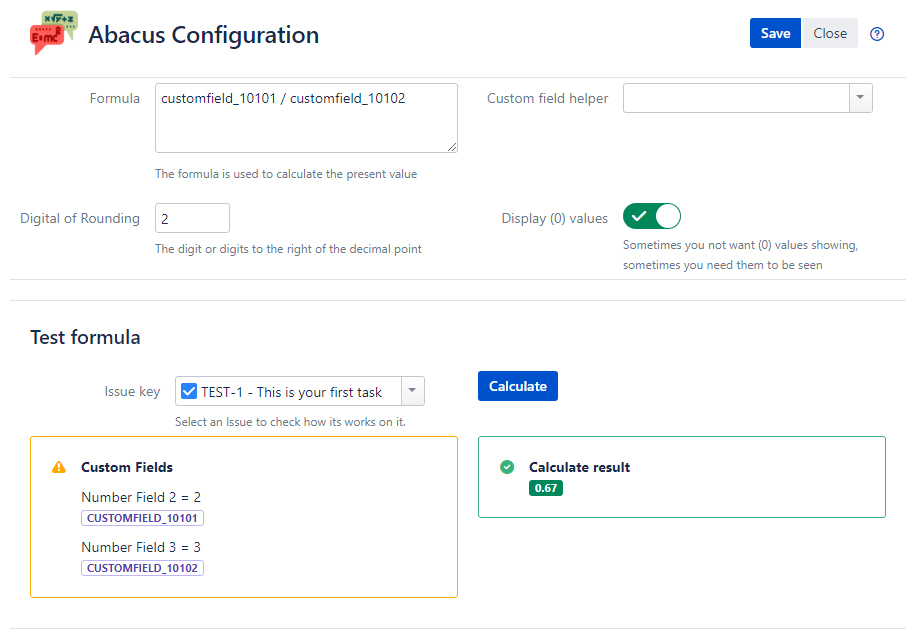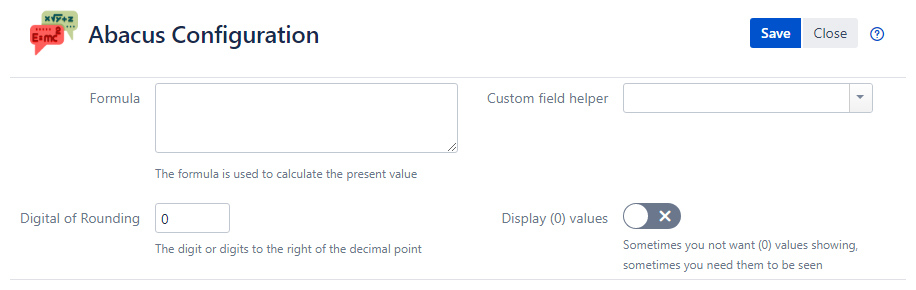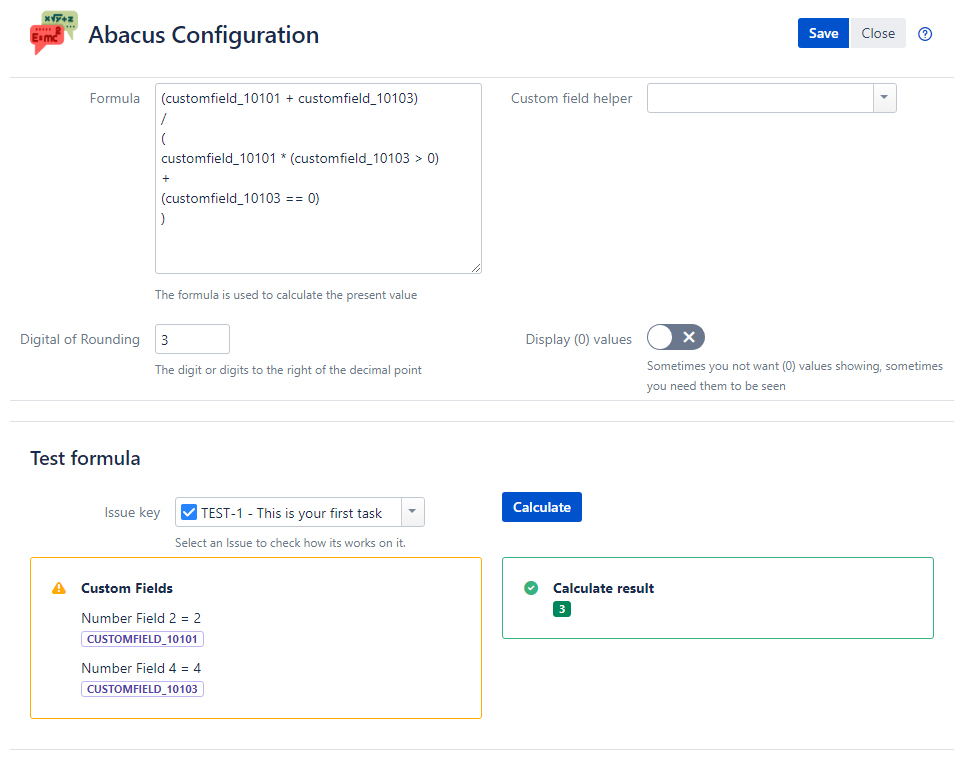# Abacus### Description

Abacus is a calculating tool developed by UX Software especially for providing easy way to calculate math operations.

There are no programming skills required, just create Abacus fields and set formulas inside.

### Features

• Abacus Fields work well with fields that use numeric search templates.
• Abacus Fields are able to quickly add, divide, multiply and subtract, sine, cosine, tangent in complex or easy computing’s.
• Abacus Fields are searchable, - so you can create filters abased on it.
• Abacus Fields coming with rounding, - you can choose 0-3 symbols.
• Abacus Fields can be exported in Excel via standard JIRA features.
• Abacus Fields can calculate Totals!
• Select an Issue to check how its works on it.### Use Cases

Abacus is useful tool for calculations within the Financial, Sales and Investing departments. Calculate Revenue, Costs, Effective Annual Rates and Totals.

Money management is an art which includes saving the right amounts and investing in the right instruments. However, there are several factors such as inflation and time that lower the value of money. Therefore, it is necessary to learn how to calculate the worth of one's investments.

Story Points and Velocity in Agile Management. Velocity measures the number of 'estimation units' that a team usually completes from sprint to sprint. It is effectively a productivity rate based on an estimation of volume of work, and it is best worked out in a measure other than 'time'.

### Management

Easy to create, edit and delete fields.
Configuration menu is available from Custom Fields.### Operators

All common arithmetic operators are supported. Boolean operators are also fully supported. Boolean expressions are evaluated to be either 1 or 0 (true or false respectively).

• Addition, Subtraction -+
• Multiplication *
• Division /
• Power ^
• Modulus %
• The number π (3.14159...) pi()
• The number e (2.71828...)e()
• Less or Equal, More or Equal >=<=
• Less Than, Greater Than ><
• Not Equal, Equal ==!=
• Boolean And &&
• Boolean Or ||
• Boolean Not !

### Functions

• Sinesin()
• Cosinecos()
• Tangenttan()
• Arc Sineasin()
• Arc Cosineacos()
• Arc Tangentatan()
• Hyperbolic Sinesinh()
• Hyperbolic Cosinecosh()
• Hyperbolic Tangenttanh()
• Inverse Hyperbolic Sineasinh()
• Inverse Hyperbolic Cosineacosh()
• Inverse Hyperbolic Tangentatanh()
• Natural Logarithmln()
• Logarithm base 10log()
• Angleangle()
• Absolute Value / Magnitudeabs()
• Random number (between 0 and 1)rand()
• Modulusmod()
• Square Rootsqrt()
• Sumsum()

### Example

• If you need to sum a custom field in issue sum(customfield_10001,customfield_10002)
• If you need to use logic.
Logic returns 1 if true or 0 if false customfield_10103 > 0
###### Formula
``` `(customfield_10101 + customfield_10102) / ( customfield_10101 * (customfield_10103 > 0) +  (customfield_10103 == 0) )`
```
###### How is calculated
``` ```(2 + 4) / (2 * (4 > 0) + (4 == 0)) - Fill custom field value
(2 + 4) / (2 * 1 + 0) - Calculates logic
6 / 2 - Calculates the value``` ```
•### Pricing

10 users
\$5/year
• UX Software: Abacus for Jira Core (Server) 10 Users: Commercial License
• 12 Months Valid
• \$10 First Year
25 users
\$27.5/year
• UX Software: Abacus for Jira Core (Server) 25 Users: Commercial License
• 12 Months Valid
• \$55 First Year
50 users
\$75/year
• UX Software: Abacus for Jira Core (Server) 50 Users: Commercial License
• 12 Months Valid
• \$150 First Year
100 users
\$125/year
• UX Software: Abacus for Jira Core (Server) 100 Users: Commercial License
• 12 Months Valid
• \$250 First Year